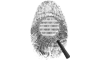## Diffie-Hellman Key Exchange

### STEP 1A

###### Select a prime number (p) and a number for generator (α), where (α) is the lowest primitive root of the prime number (p). You can generate and test primitive roots at our Primitive Root Calculator.
Prime Number (p):
Generator Number (α):

### STEP 1B

###### Input a value for ALICE and BOB. This can be any value you pick but it should be something random. These values will be used as the private keys for Alice and Bob.
ALICE's Private Number (XA):
BOB's Private Number (XB):

### STEP 2: GENERATE Secret Keys:

###### Alice and Bob exchange or publish their public keys.
ALICE's Public Key:
BOB's Public Key: